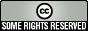# News You Can Bruise for 2007September 6

This modelling led to the discovery that all the French classical sauces belong to 23 groups only: W, O, W/S, O/W, S/W, (O+S)/W, (W/S)/W, O+(W/S), (G+O)/W, (G+O+S)/W, (O+(W/S))/W, (S+(W/S))/W, ((W+S)/O)/S, (O+S+(W/S))/W, ((W/S)+ (W⊃S))/W, (O + (W/S)/W)/S, ((O+(W/S))/W)/S, (O/W) + ((G+O)/W), (O+(W/S)+(W⊃S))/W, (S+(W/S)+(W⊃S))/W, (((W/S)+(W⊃S))/W)/S, (O+S+(W/S)+(W⊃S))/W, (O+S+((G+O)/W))/W.

[Main]Unless otherwise noted, all content licensed by Leonard Richardsonunder a Creative Commons License.